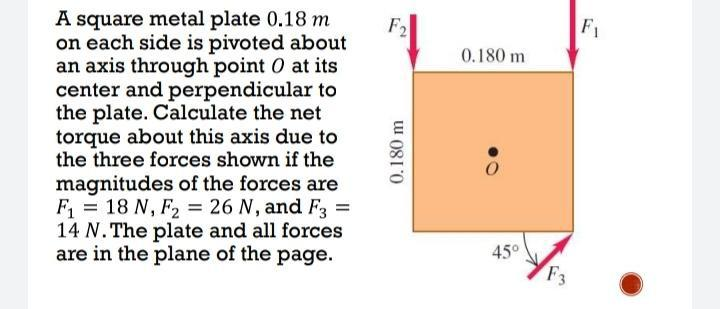Question:

# F2 F 0.180 m A square metal plate 0.18 m on each side is pivoted about an axis through point 0 at its center and perpendicular tF2 F 0.180 m A square metal plate 0.18 m on each side is pivoted about an axis through point 0 at its center and perpendicular to the plate. Calculate the net torque about this axis due to the three forces shown if the magnitudes of the forces are Fi = 18 N, F2 = 26 N, and F3 14 N. The plate and all forces are in the plane of the page. 0.180 m 45° F3